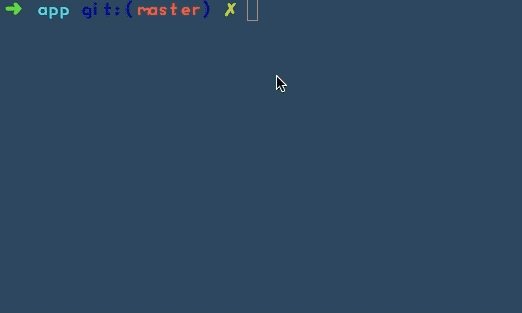### 引言

Observables can "return" multiple values over time

Rxjs中一个普通的Obseravle对象，赋值时间点（next）之后的订阅才是有效的！当然BehaviorObservable会有一个当前值，任何时候的一个订阅都会立即收到当前值、执行消费函数（Observer）

### 定义类

``````function Observable() {
this._observers = [];
this._val = null;
}``````

### 定义next方法

``````Observable.prototype.next = function (val) {
this._val = val
var _observer = null
for(var i in this._observers) {
_observer = this._observers[i]
if (!_observer) continue
Promise.resolve(val).then(_observer)
}
}
``````

### 定义subscribe方法

``````Observable.prototype.subscribe = function (observer) {
if (typeof observer !== 'function') return
Promise.resolve(this._val).then(observer)
this._observers.push(observer)
}``````

### 添加map方法

``````Observable.prototype.map = function (project) {
var obs = new Observable()
this.subscribe(function(v) {
obs.next(project(v))
})
return obs
}``````

### 使用

``````const Observable = require('./Observable.class')

const obs = new Observable()
obs.next('99')

obs.subscribe((v) => {
console.log(v)
})

obs.next('100')
obs.next('101')``````

### 结果